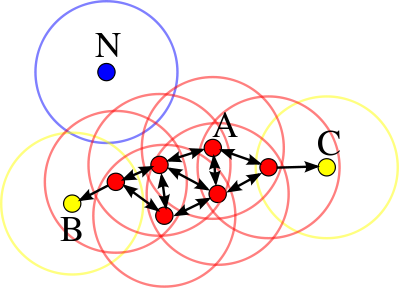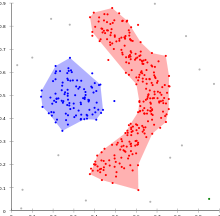# DBSCAN

DBSCAN

DBSCAN (for density-based spatial clustering of applications with noise) is a data clustering algorithm proposed by Martin Ester, Hans-Peter Kriegel, Jörg Sander and Xiaowei Xu in 1996. It is a density-based clustering algorithm because it finds a number of clusters starting from the estimated density distribution of corresponding nodes. DBSCAN is one of the most common clustering algorithms and also most cited in scientific literature. OPTICS can be seen as a generalization of DBSCAN to multiple ranges, effectively replacing the ε parameter with a maximum search radius.

## Basic ideaPoints at A are core points. Points B and C are density-reachable from A and thus density-connected and belong to the same cluster. Point N is a noise point that is neither a core point nor density-reachable. (MinPts=3 or MinPts=4)

DBSCAN's definition of a cluster is based on the notion of density reachability. Basically, a point q is directly density-reachable from a point p if it is not farther away than a given distance ε (i.e., is part of its ε-neighborhood), and if p is surrounded by sufficiently many points such that one may consider p and q be part of a cluster. q is called density-reachable (note: this is different from "directly density-reachable") from p if there is a sequence$p_1,\ldots,p_n$ of points with p1 = p and pn = q where each pi + 1 is directly density-reachable from pi. Note that the relation of density-reachable is not symmetric (since q might lie on the edge of a cluster, having insufficiently many neighbors to count as a genuine cluster element), so the notion of density-connected is introduced: two points p and q are density-connected if there is a point o such that both p and q are density reachable from o.

A cluster, which is a subset of the points of the database, satisfies two properties:

1. All points within the cluster are mutually density-connected.
2. If a point is density-connected to any point of the cluster, it is part of the cluster as well.

## Algorithm

DBSCAN requires two parameters: ε (eps) and the minimum number of points required to form a cluster (minPts). It starts with an arbitrary starting point that has not been visited. This point's ε-neighborhood is retrieved, and if it contains sufficiently many points, a cluster is started. Otherwise, the point is labeled as noise. Note that this point might later be found in a sufficiently sized ε-environment of a different point and hence be made part of a cluster.

If a point is found to be part of a cluster, its ε-neighborhood is also part of that cluster. Hence, all points that are found within the ε-neighborhood are added, as is their own ε-neighborhood. This process continues until the cluster is completely found. Then, a new unvisited point is retrieved and processed, leading to the discovery of a further cluster or noise.

## Pseudocode

DBSCAN(D, eps, MinPts)
C = 0
for each unvisited point P in dataset D
mark P as visited
N = regionQuery(P, eps)
if sizeof(N) < MinPts
mark P as NOISE
else
C = next cluster
expandCluster(P, N, C, eps, MinPts)

expandCluster(P, N, C, eps, MinPts)
for each point P' in N
if P' is not visited
mark P' as visited
N' = regionQuery(P', eps)
if sizeof(N') >= MinPts
N = N joined with N'
if P' is not yet member of any cluster


## Complexity

DBSCAN visits each point of the database, possibly multiple times (e.g., as candidates to different clusters). For practical considerations, however, the time complexity is mostly governed by the number of regionQuery invocations. DBSCAN executes exactly one such query for each point, and if an indexing structure is used that executes such a neighborhood query in O(log n), an overall runtime complexity of$O(n \cdot \log n)$ is obtained. Without the use of an accelerating index structure, the run time complexity is O(n2). Often the distance matrix of size (n2n) / 2 is materialized to avoid distance recomputations. This however also needs O(n2) memory.DBSCAN can find non-linearly separable clusters. This dataset cannot be adequately clustered with k-means or EM clustering.

1. DBSCAN does not require you to know the number of clusters in the data a priori, as opposed to k-means.
2. DBSCAN can find arbitrarily shaped clusters. It can even find clusters completely surrounded by (but not connected to) a different cluster. Due to the MinPts parameter, the so-called single-link effect (different clusters being connected by a thin line of points) is reduced.
3. DBSCAN has a notion of noise.
4. DBSCAN requires just two parameters and is mostly insensitive to the ordering of the points in the database. (Only points sitting on the edge of two different clusters might swap cluster membership if the ordering of the points is changed, and the cluster assignment is unique only up to isomorphism.)

1. DBSCAN can only result in a good clustering as good as its distance measure is in the function regionQuery(P,ε). The most common distance metric used is the euclidean distance measure. Especially for high-dimensional data, this distance metric can be rendered almost useless due to the so called "Curse of dimensionality", rendering it hard to find an appropriate value for ε. This effect however is present also in any other algorithm based on the euclidean distance.
2. DBSCAN cannot cluster data sets well with large differences in densities, since the minPts-ε combination cannot be chosen appropriately for all clusters then.

See the section on extensions below for algorithmic modifications to handle these issues.

## Parameter estimation

Every data mining task has the problem of parameters. Every parameter influences the algorithm in specific ways. For DBSCAN the parameters ε and MinPts are needed. The parameters must be specified by the user of the algorithms since other data sets and other questions require different parameters. An initial value for ε can be determined by a k-distance graph. As a rule of thumb, k can be derived from the number of dimensions in the data set D as$k\geq D+1$. However, larger values are usually better for data sets with noise.

Although this parameter estimation gives a sufficient initial parameter set the resulting clustering can turn out to be not the expected partitioning. Therefore research has been performed on incrementally optimizing the parameters against a specific target value.

OPTICS can be seen as a generalization of DBSCAN that replaces the ε parameter with a maximum value that mostly effects performance. MinPts then essentially becomes the minimum cluster size to find. While the algorithm is a lot easier to parameterize then DBSCAN, the results are a bit more difficult to use, as it will usually produce a hierarchical clustering instead of the simple data partitioning that DBSCAN produces.

## Generalization

Generalized DBSCAN or GDBSCAN  is a generalization by the same authors to arbitrary "neighborhood" and "dense" predicates. The ε and minpts parameters are removed from the original algorithm and moved to the predicates. For example on polygon data, the "neighborhood" could be any intersecting polygon, whereas the density predicate uses the polygon areas instead of just the object count.

## Extensions

The basic DBSCAN algorithm has been used as a base for many other developments, such as parallelisation by Domenica Arlia and Massimo Coppola or an enhancement of the data set background to support uncertain data presented by Dirk Habich and Peter Benjamin Volk. The basic idea has been extended to hierarchical clustering by the OPTICS algorithm. DBSCAN is also used as part of subspace clustering algorithms like PreDeCon and SUBCLU.

## Availability

An example implementation of DBSCAN is available in the ELKI framework. Notice that this implementation is not optimized for speed but for extensibility. Thus, this implementation can use various index structures for sub-quadratic performance and supports various distance functions and arbitrary data types, but it may be outperformed by low-level optimized implementations on small data sets.

Wikimedia Foundation. 2010.

### Look at other dictionaries:

• DBSCAN — (Density Based Spatial Clustering of Applications with Noise, etwa: Dichtebasierte räumliche Clusteranalyse mit Rauschen) ist ein von Martin Ester, Hans Peter Kriegel, Jörg Sander und Xiaowei Xu entwickelter Data Mining Algorithmus zur… …   Deutsch Wikipedia

• OPTICS algorithm — OPTICS ( Ordering Points To Identify the Clustering Structure ) is an algorithm for finding density based clusters in spatial data. It was presented by Mihael Ankerst, Markus M. Breunig, Hans Peter Kriegel and Jörg Sander. Its basic idea is… …   Wikipedia

• Cluster analysis — The result of a cluster analysis shown as the coloring of the squares into three clusters. Cluster analysis or clustering is the task of assigning a set of objects into groups (called clusters) so that the objects in the same cluster are more… …   Wikipedia

• OPTICS — (Ordering Points To Identify the Clustering Structure, etwa ‚Punkte ordnen um die Clusterstruktur zu identifizieren‘) ist ein dichtebasierter Algorithmus zur Clusteranalyse. Er wurde von Mihael Ankerst, Markus M. Breunig, Hans Peter Kriegel und… …   Deutsch Wikipedia

• Clusteranalyse — Dieser Artikel wurde aufgrund von inhaltlichen Mängeln auf der Qualitätssicherungsseite der Redaktion Informatik eingetragen. Dies geschieht, um die Qualität der Artikel aus dem Themengebiet Informatik auf ein akzeptables Niveau zu bringen. Hilf… …   Deutsch Wikipedia

• Data-Mining — Unter Data Mining (der englische Begriff bedeutet etwa „aus einem Datenberg etwas Wertvolles extrahieren“, eine adäquate deutsche Übersetzung existiert nicht. Der Duden empfiehlt die Schreibweise „Data Mining“) versteht man die… …   Deutsch Wikipedia

• Zeitkomplexität — Unter der Zeitkomplexität eines Problems wird in der Datenverarbeitung die Anzahl der Rechenschritte verstanden, die ein optimaler Algorithmus zur Lösung dieses Problems benötigt, in Abhängigkeit von der Länge der Eingabe. Dabei wird hier auch… …   Deutsch Wikipedia

• Cluster (Informatik) — Als Cluster bezeichnet man in der Informatik und Statistik eine Gruppe von Datenobjekten mit ähnlichen Eigenschaften. Die Menge der in einem Datensatz gefundenen Cluster bezeichnet man als Clustering, Verfahren zur Berechnung einer solchen… …   Deutsch Wikipedia

• k-Means-Algorithmus — Ein k Means Algorithmus ist ein Verfahren zur Clusteranalyse. Dabei wird aus einer Menge von ähnlichen Objekten eine vorher bekannte Anzahl von k Gruppen gebildet. Der Algorithmus ist eine der am häufigsten verwendeten Techniken zur Gruppierung… …   Deutsch Wikipedia

• Liste von Algorithmen — Dies ist eine Liste von Artikeln zu Algorithmen in der deutschsprachigen Wikipedia. Siehe auch unter Datenstruktur für eine Liste von Datenstrukturen. Inhaltsverzeichnis 1 Klassen von Algorithmen nach Komplexität 2 Klassen von Algorithmen nach… …   Deutsch Wikipedia